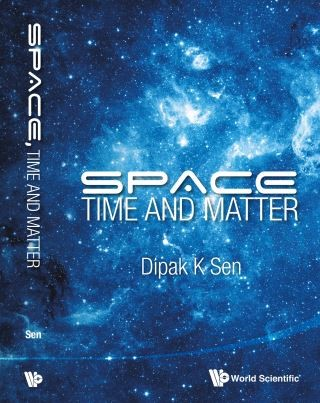## Space, Time And Matter• Description
• Author
• Info
• Reviews

### Description

This volume deals with the fundamental concepts of space, time and matter. It presents a novel reformulation of both the special and general theory of relativity, in which time does not constitute the fourth dimension in a conventional 4-dimensional space-time. Instead, the role of time is played by the flow of a vector field on a 3-dimensional space. The standard models of de Sitter, Schwarzschild and Kerr space-times are reformulated in a purely 3-dimensional manifold.

The volume also presents a theory of matter in which the fundamental particles, such as baryons and leptons, appear as a result of an interaction between left-handed and right-handed 2-component Weyl neutrinos. The Appendices contain a comprehensive treatment of classical mechanics in terms of Hamiltonian vector fields on symplectic manifolds. Graduate students of mathematical physics or theoretical physics, as well as academics, will find this volume of interest.

Contents:
• Space and Time:
• Introduction
• The Hyperbolic Structure of the Space
• Relativistic Kinematics on 3-Manifolds
• Class of Inertial Observers and Generalized Lorentz Matrices
• Classical Relativistic Field Dynamics
• Relativistic Field Dynamics on 3-Manifolds
• Flat-Space Solutions
• The de Sitter Solution
• A New Solution of the Vacuum Einstein Field Equations
• A Solution of Mathematical Interest
• The Schwarzschild Solution
• From Space-Time 4-Metric to 3-Metric and 3-Vector Field
• The Kerr Solution
• The Maxwell Equations
• Matter:
• Introduction
• The Photon and the Weyl Neutrinos
• A Neutrino Theory of Matter